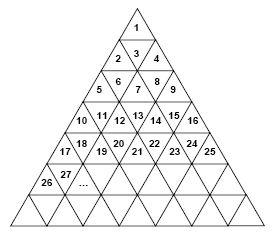Competitions

# Triangle Trouble

Time limit 1 second
Memory limit 64 MiB

Consider a triangle of infinite size divided into smaller triangles numbered as shown in the following figure. Two of these smaller triangles are adjacent if they share a common border (not just a common point). Since each triangle has three sides, a triangle is adjacent to at most three other triangles. Triangles that are adjacent to two or fewer other triangles are on the border of the infinite triangle.Given a triangle’s number, it is relatively simple to visually determine the numbers of the triangles that are adjacent to it. For example, if we are given number 13, the triangles that are adjacent to it are 712, and 14. If we pick a border triangle, such as 25, we can still easily determine that the only triangles that are adjacent to it are 24 and 35. Your task is to write a program that can perform this function.

## Input data

The first line of the input contains an integer n that represents the number of data collections that follow where each data collection is on a single line. Each data collection contains a single integer value representing the number of a triangle.

The value of n will be between 1 and 100, inclusive. The number of each input triangle will be between 1 and 1000000, inclusive.

## Output data

Your program should produce n lines of output (one for each data collection). The output for each data collection should be the numbers of the triangles that are adjacent to the input triangle. The list should be presented in ascending sorted order. There should be a single space between each pair of triangle numbers.

The output is to be formatted exactly like that for the sample output given below.

## Examples

Input example #1
3
13
25
1

Output example #1
7 12 14
24 35
3

Source TCEA State Programming Contest April, 2008